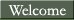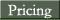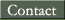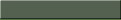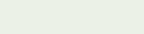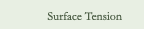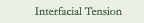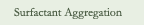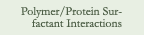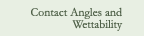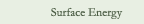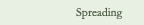Surface Tension is the amount of work necessary to create a unit area of air/liquid interface (mN/m = mJ/m2 = dynes/cm)

It varies from liquid to liquid due to the strength of the interactive properties between the molecules which make up the liquid (i.e. how much the molecules of the liquid resist being at a surface, relative to being in the bulk, where they have associations with other like molecules).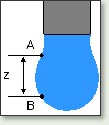Surface tension measurements can be performed either by Wilhelmy Plate Method, by Pendant Drop Method, or by the Bubble Pressure Technique.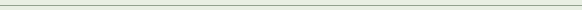Interfacial Tension is the amount of work necessary to create a unit area of liquid/liquid interface (mN/m = mJ/m2 = dynes/cm).

Interfacial tensions have an imbalance of intermolecular forces, like surface tension, but usually to a lesser degree. The nterfacial tension between two liquids generally falls in between the two pure liquid surface tensions.

Interfacial tension can be measured by the DuNouy Ring Method (for interfacial tensions down to approximately 4 mN/m), or preferably, by the Pendant Drop Method (for interfacial tensions down to 0.01mN/m).

Both dynamic (non-equilibrium) and static (equilibrium) interfacial tensions can be measured on samples.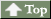Surfactant Aggregation is the tendency for surfactant particles to gather together to form a larger particle. Aggregation of surfactant molecules in solution occurs because either the head group, or tail group, is not soluble in the bulk solvent.Polymer / Protein Surfactant Interaction is a highly complex topic. Both polymer and protein interaction with surfactants can change critical micelle concentrations, either by raising or lowering them.Contact Angles and Wettability are interrelated components. The angle of incidence between a solid surface and a liquid (in degrees) can predict how wettable, or non-wettable the solid is with that particular liquid. Generally the higher the contact angle with the liquid, the less wettable the solid. If water is used as the test liquid, this can also give insight into how hydrophobic or hydrophilic the solid in question. While this technique can give an answer for a single liquid and solid, a characterization of the solid by determining the surface energy is a better method for understanding the surface properties of the solid.Surface Energy is the determination of the amount of energy which exists in a unit area of air/solid interface (mJ/m2). There are several methods available to determine surface energy, some relying on one liquid (like Zisman -one component - low surface energy solid with little or no polarity ), some on multiple liquids ( like Owens-Wendt -two component -developed to account for polar interactions between solids and liquids), some on two liquids (like Fowkes-two component also and mathematically equivalent to Owens-Wendt describing polar and non-polar interactions), and some to delve into higher interactions (like vanOss - three component - developed to characterize the acid and base components of the polar component). An important feature to to remember about solid surface energy analysis is it is improper, and perhaps even useless, to compare any two surface energy values that were not determined in the same manner.Spreading is what happens when a liquid already in contact with a solid spreads. The spreading coefficient (S) is a widely used means of characterizing spreading problems with one overall value. It is defined thermodynamically as follows:

S = s S - s SL - s L

where s S = the surface energy of the solid, s SL = the interfacial tension between your spreading liquid and the solid, and s L = the surface tension of the spreading liquid.

And, the general rules of thumb are:

If S is calculated to be less than 0, then no spreading will occur.

If S is calculated to be between 0 and 10, then spreading will occur to an extent related to the value of S. The higher S is, the greater the extent of spreading.

If S is greater than 10, complete spreading will occur.